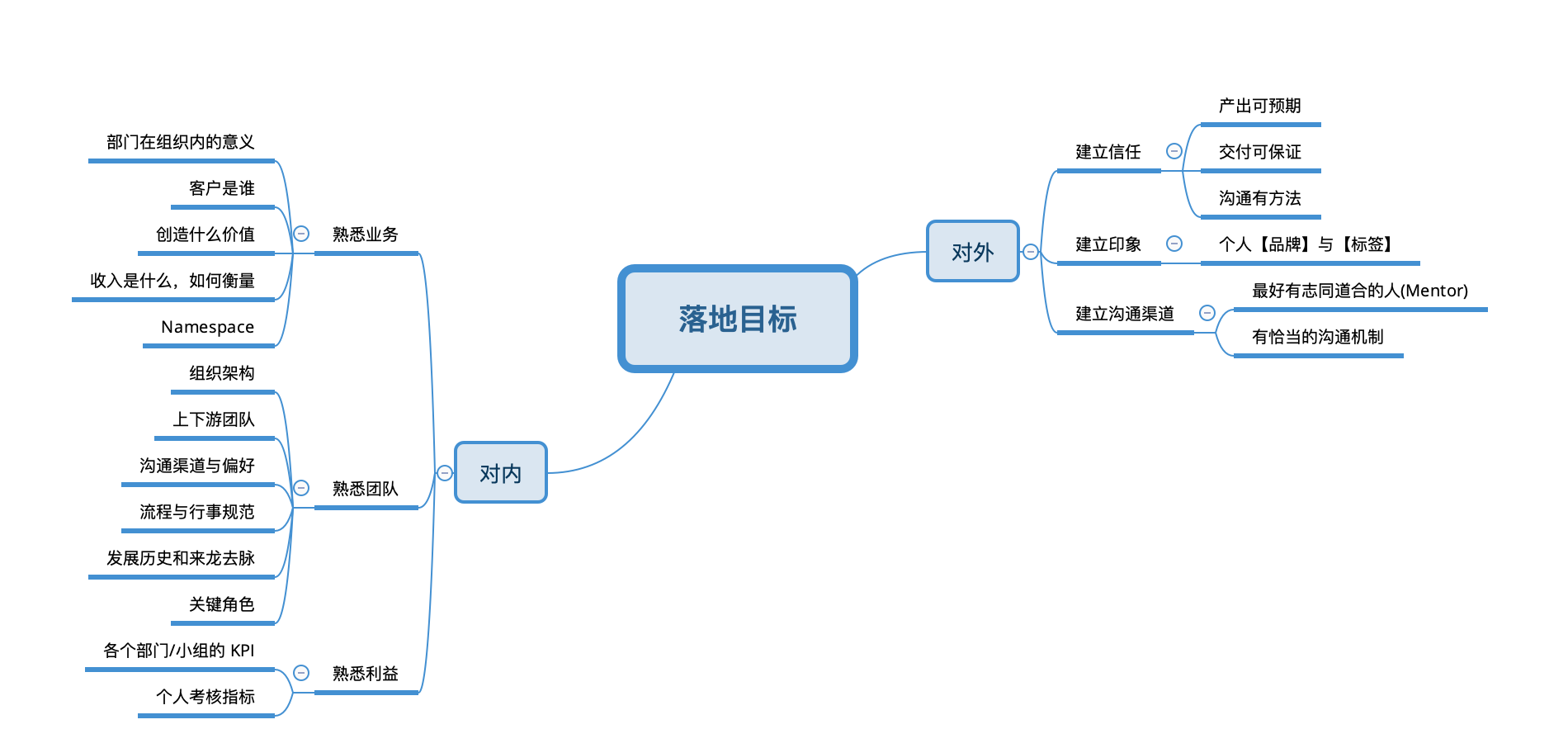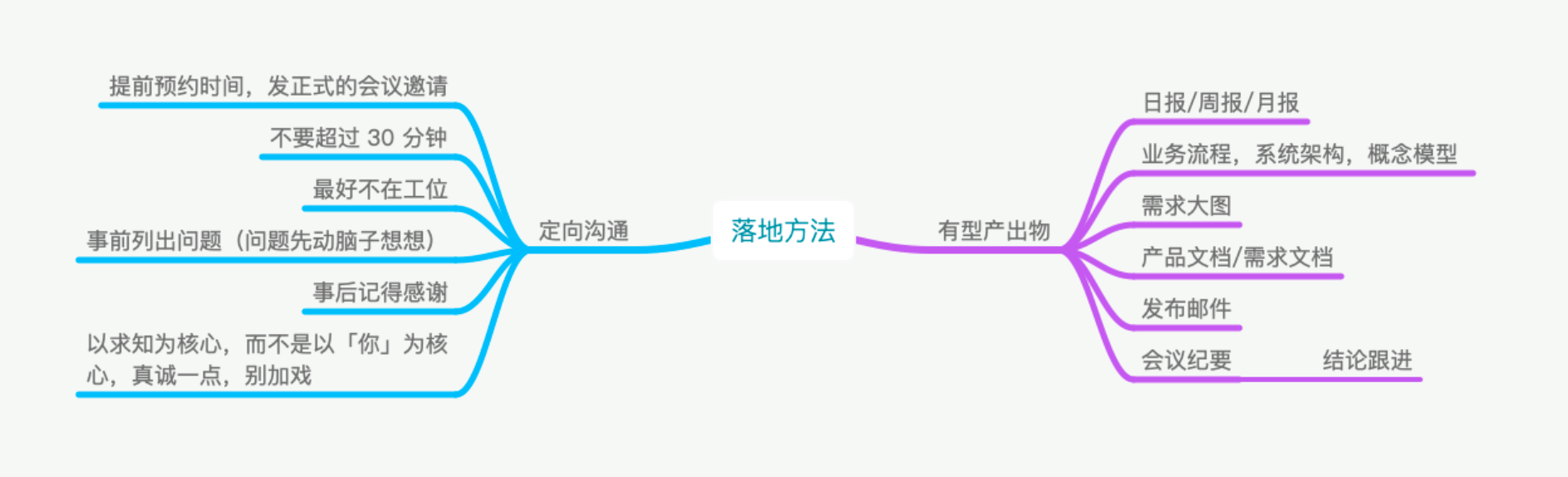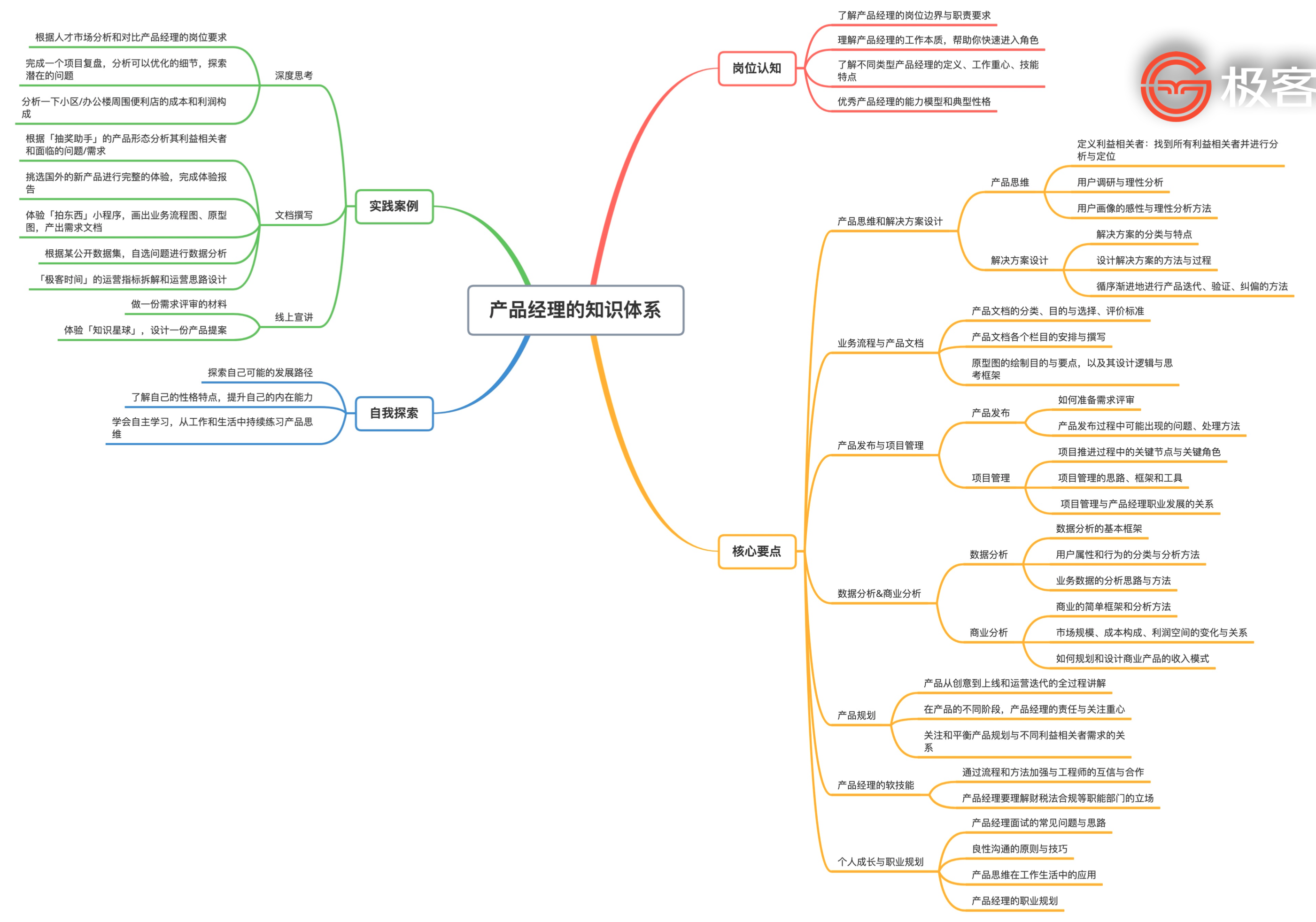算法：罗马数字转换为整数，RxSwift 的好处，git pull 问题解决 error: cannot lock ref，产品经理新人如何落地 John 易筋 ARTS 打卡 Week 34John(易筋)

1. Algorithm: 每周至少做一个 LeetCode 的算法题

LeetCode 全集请参考：LeetCode Github 大全

算法

Roman numerals are represented by seven different symbols: I, V, X, L, C, D and M.

Symbol       ValueI             1V             5X             10L             50C             100D             500M             1000

For example, 2 is written as II in Roman numeral, just two one's added together. 12 is written as XII, which is simply X + II. The number 27 is written as XXVII, which is XX + V + II.

Roman numerals are usually written largest to smallest from left to right. However, the numeral for four is not IIII. Instead, the number four is written as IV. Because the one is before the five we subtract it making four. The same principle applies to the number nine, which is written as IX. There are six instances where subtraction is used:

• I can be placed before V (5) and X (10) to make 4 and 9.

• X can be placed before L (50) and C (100) to make 40 and 90.

• C can be placed before D (500) and M (1000) to make 400 and 900.

Given a roman numeral, convert it to an integer.

Example 1:

Input: s = "III"Output: 3

Example 2:

Input: s = "IV"Output: 4

Example 3:

Input: s = "IX"Output: 9

Example 4:

Input: s = "LVIII"Output: 58Explanation: L = 50, V= 5, III = 3.

Example 5:

Input: s = "MCMXCIV"Output: 1994Explanation: M = 1000, CM = 900, XC = 90 and IV = 4.

Constraints:

1 <= s.length <= 15

s contains only the characters ('I', 'V', 'X', 'L', 'C', 'D', 'M').

It is guaranteed that s is a valid roman numeral in the range [1, 3999].

Map 记录解法

1. 先用 map 记录对应的 char 和数字。

2. 如果前一个字母比后一个字母小，则说明是减法。

3. 最后一个字母累加即可。

class Solution {    public int romanToInt(String s) {        Map<Character, Integer> map = new HashMap<>();        map.put('I', 1);        map.put('V', 5);        map.put('X', 10);        map.put('L', 50);        map.put('C', 100);        map.put('D', 500);        map.put('M', 1000);                char[] chars = s.toCharArray();                int sum = 0;        for(int i = 0; i < chars.length - 1; i++) {            if (map.get(chars[i]) < map.get(chars[i + 1])) {                sum -= map.get(chars[i]);            } else {                sum += map.get(chars[i]);            }        }        sum += map.get(chars[chars.length - 1]);                return sum;    }}

2. Review: 阅读并点评至少一篇英文技术文章

Why should we use RxSwift

1. 请求时候后重试；

2. 全局通知、button 事件等可以优雅在一个链式事件里面；

3. 多个请求的前后依赖管理；

4. KVO 优雅实现

好处

• 可组合<-因为 Rx 是组合的昵称

• 可重用<-因为可组合

• 声明性<-因为定义是不可变的，并且仅数据发生更改

• 可理解，简洁<-提升抽象水平并消除瞬态

• 稳定<-因为 Rx 代码已经过全面的单元测试

• 状态较少-因为将应用程序建模为单向数据流

• 没有泄漏<-因为资源管理容易

问题

git pull的时候报错error: cannot lock ref

解决方案

git remote prune origin

说明• 产品信任有效落地

• 产品经理落地的目标

• 产品新人的落地态度

• 具体的工具和方法

• 产品训练营大纲

平稳落地很重要

• 针对一线战斗型产品经理，其它岗位可能也使用；

• 首因效应（Primacy Effect）；

• 互联网公司贴标签的速度非常快，撕标签的速度非常慢；

• 新手红利期对产品尤其重要

产品经理落地的目标• 熟悉业务：信任用在 3 个月内问各种不懂的问题。如果超过太长时间，如果对业务还不熟悉，会被贴业务能力差的标签。

• 建立信任的重要性：如果所有事情都公事公办，很难做成大事。所以互相信任很重要。

• 熟悉利益：努力成为 Giver，事情更好往前推动，帮助到别人，自己也容易获得收益。

产品经理落地的工作态度

• 严于律己，宽于待人。

• 确定自己的风格和边界（标签）。

• 不要傲慢，存在即合【理】，找出这个【理】，但不一定认同它。

• 不提当年勇，不要侃侃而谈。

• 集中快速有效解决所有【蠢问题】。

产品信任落地的方法和手段

• 个体决策与群体决策的差异。

* 市场规模越大，越偏向于 To C，市场规模越小，越偏向于服务业。

* 法人不是人，不能想当然。

方法和手段极客大学产品经理训练营大纲评论